# Electronics and Communication Engineering - Exam Questions Papers

16.

Find 'X' in the circuit below :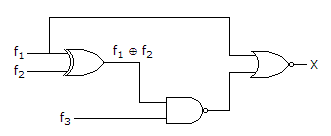f1(A, B, C, D) = Σ(6, 7, 13, 14);
f2(A, B, C, D) = Σ(3, 6, 7);
f3(A, B, C, D) = Σ(5, 6, 7, 14, 15)

 A. p(0, 1, 2, 3, 4, 5, 6, 7, 8, 9, 10, 11, 12, 13, 15) B. 0 C. Σ(14) D. 1

Answer: Option C

Explanation:

f1(A, B, C, D) = ∑(6, 7, 13, 14)

f2(A, B, C, D) = ∑(3, 6, 7)

f1f2 = ∑(3, 13, 14)

f3 x (f1f2) = ∑(14) = y

X = f1 x y ⇒ ∑14.

17.

Suppose that the modulating signal is m(t) = 2cos (2pfmt) and the carrier signal is xC(t) = AC cos(2pfct), which one of the following is a conventional AM signal without over modulation?

 A. x(t) = Acm(t)cos(2pfct) B. x(t) = Ac [1 + m(t)]cos (2pfct) C.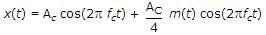D. x(t) = Accos(2pfmt)cos(2pfct) + Acsin(2pfmt)sin(2pfct)

Answer: Option C

Explanation: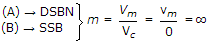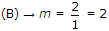(C) is without over modulation.

18.

What are the values of Emax and Emin displayed on the oscilloscope, when a 1 kV P-P carries is modulated to 50%?

 A. 2 kV, 0.5 kV B. 1 kV, 0.5 kV C. 0.75 kV, 0.25 kV D. 0.5 kV, 1.5 kV

Answer: Option C

Explanation: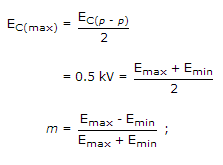Emax - Emin = 0.5 x 1 kV = 0.5 kV

2Emax = 1.5 kVi, Emax = 0.75 kV

Emin = 0.25 kV.

19.

Consider the common emitter amplifier shown below with the following circuit parameters: β = 100, gm = 0.3861 A/V, r0 = ∞ rp = 259 Ω, Rs = 1 kΩ, RB = 93 kΩ, RC = 250 Ω, RL = 1 kW, C1 = ∞ and C2 = 4.7mF.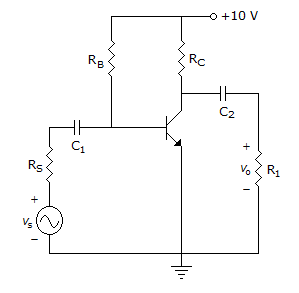The resistance seen by the source Vs is

 A. 258 Ω B. 1258 Ω C. 93 kΩ D. ∞

Answer: Option B

Explanation:

Zs = Rs + (RB || Brs)

rc = 2.475 = 1.258 kV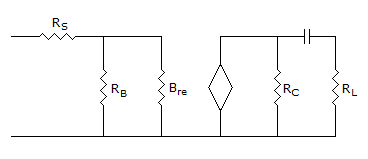20.

As the temperature increases the mobility of electrons __________ .

 A. decreases because the number of carries increases with increased collisions B. increases because the number of carries decreases with decreased collisions C. remains same D. none of the above

Answer: Option A

Explanation:

Mobility of electrons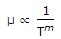m = 2.5 for electrons and 2.7 for holes in Si.

#### Current Affairs 2021

Interview Questions and Answers